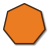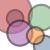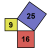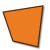Games
Problems
Go Pro!

# Geometry

Reference > Mathematics > Geometry

# Featured ReferenceThis unit is a follow-up to my Quadrilaterals unit, but explores figures with more than four sides.A unit on circles, arcs, chords, secants, and tangents. This reference unit is just problems, without readings.Using the Pythagorean Theorem to find the sides of a right triangleA unit describing the relationships of sides, angles, and diagonals of various quadrilateralsRecognizing and working with similar triangles.

# Full Directory Listing

Circles, Polygons, Quadrilaterals, Similar Triangles, The Pythagorean TheoremLike us on Facebook to get updates about new resources